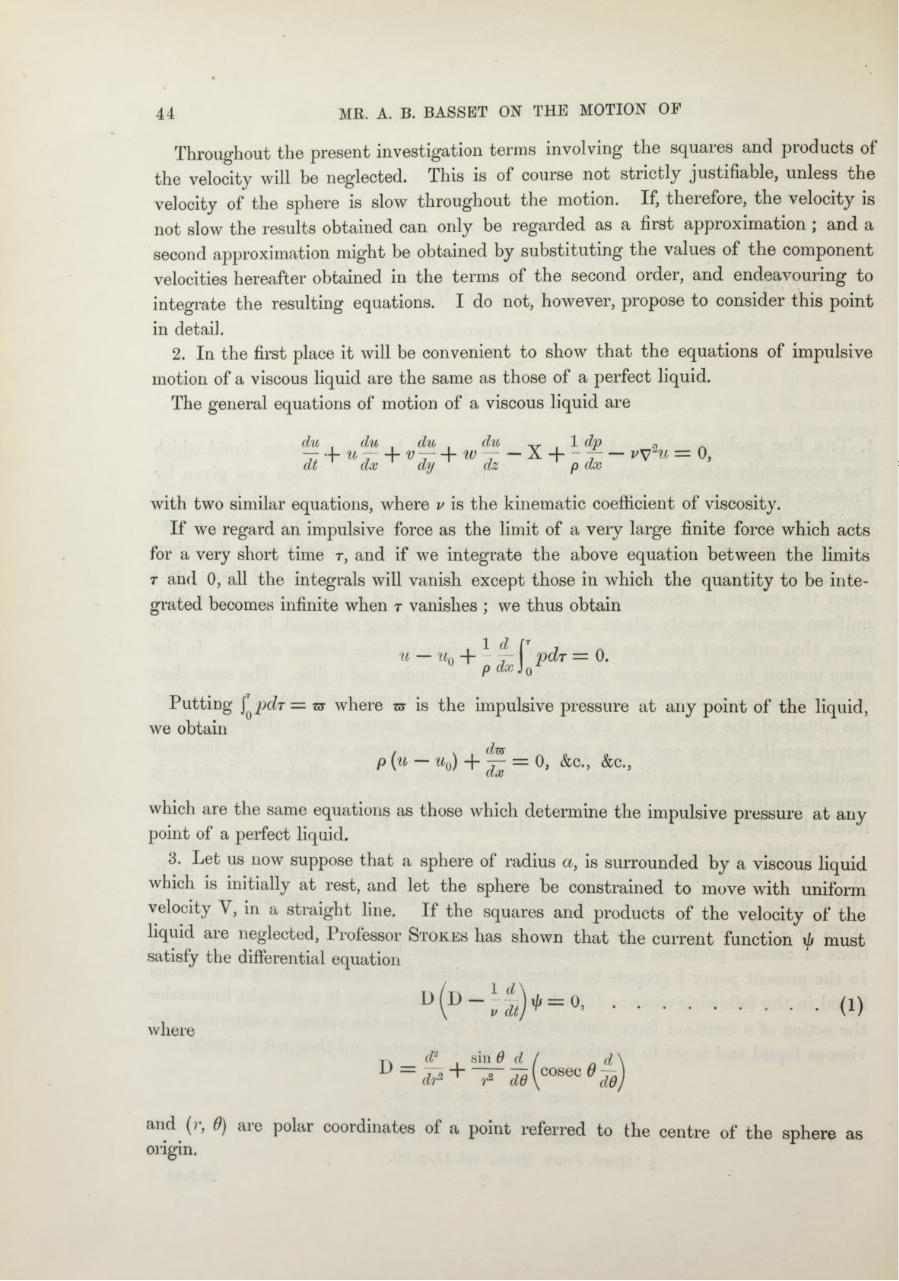# PDF Archive

Easily share your PDF documents with your contacts, on the Web and Social Networks.

## A. B. Basset. On the motion of a sphere in a viscous liquid.pdfPage 1 23421

#### Text preview

44

MR. A. B. BASSET ON THE MOTION OF

Throughout the present investigation terras involving the squares and products of
the velocity will be neglected, th is is of course not stiictly justifiable, unless the
velocity of the sphere is slow throughout the motion. If, therefore, the velocity is
not slow the results obtained can only be regarded as a fiist approximation \ and a
second approximation might be obtained by substituting the values of the component
velocities hereafter obtained in the terms of the second order, and endeavouring to
integrate the resulting equations. I do not, however, propose to consider this point
in detail.
2.
In the first place it will be convenient to show th a t the equations of impulsive
motion of a viscous liquid are the same as those of a perfect liquid.
The general equations of motion of a viscous liquid are
du,

du
It + “ * +

, du ,dn
w* ~ x +

, r , 1 dp

P&lt;

with two similar equations, where v is the kinematic coefficient of viscosity.
If we regard an impulsive force as the limit of a very large finite force which acts
for a very short time r, and if we integrate the above equation between the limits
r and 0, all the integrals will vanish except those in which the quantity to be inte­
grated becomes infinite when r vanishes ; we thus obtain
.

Putting f p d r = nr where
we obtain

1

d (r7

is the impulsive pressure a t any point of the liquid,

p (u—

u0)+ — — 0, &amp;c., &amp;c.,

which are the same equations as those which determine the impulsive pressure at any
point of a perfect liquid.
3.
Let us now suppose that a sphere of radius a, is surrounded by a viscous liquid
which is initially at rest, and let the sphere be constrained to move with uniform
velocity V, in a straight line. If the squares and products of the velocity of the
liquid are neglected, Professor S tokes has shown th at the current function xb must
satisfy the differential equation
D

(i)

where
6 d
rz dd (\ cosec dd

sin

and (&gt;, 0) aie polar coordinates of a point referred to the centre of the sphere as
origin.